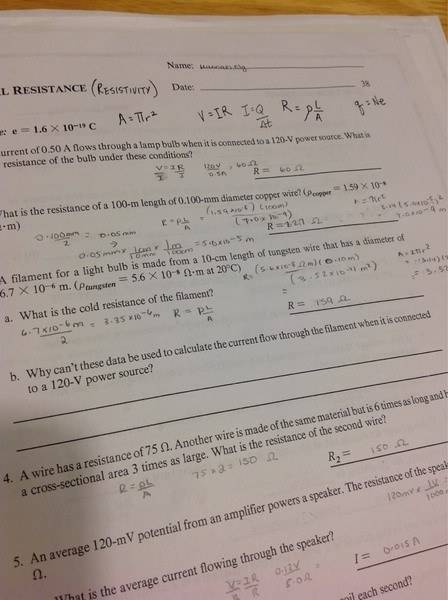# Difference between two formulas of resistance

There are two formulas for resistance
R=pl/A
V=IR

What is the difference between them?Question b on the sheet. Why can you not use that data to calculate current flow when connected to a power source. I suspect it has something to Do with the difference between these two formulas.

Last edited by a moderator:

berkeman
Mentor
There are two formulas for resistance
R=pl/A
V=IR

What is the difference between them?

View attachment 64890

Question b on the sheet. Why can you not use that data to calculate current flow when connected to a power source. I suspect it has something to Do with the difference between these two formulas.

What do the variables in those equations stand for?

When would you use the first equation to calculate resistance?

When would you use the second equation?

Last edited:
What do the variables in those equations stand for?

When would you use the first equation to calculate resistance?

When would you use the second equation?

The first formula is just talking about the resistance of a wire the second is when there's a current flowing?

berkeman
Mentor
The first formula is just talking about the resistance of a wire the second is when there's a current flowing?

You didn't answer my first question. Defining the variables will help you to determine when you use each formula...

Ok I understand it now . I know the first equation has is resistivity * length divided by area
The second is volt = current * resistance
Therefore you use the second equation when there is a current

Last edited by a moderator:
berkeman
Mentor
Ok I understand it now . I know the first equation has is resistivity * length divided by area
The second is volt = current * resistance
Therefore you use the second equation when there is a current

Yeah, the 2nd equation is Ohm's Law, and you use it when you know two of the 3 variables. The first equation is for calculating the resistance of some known geometry or volume (like a wire of known thickness, etc.).

Last edited:
nasu
Gold Member
Question b on the sheet. Why can you not use that data to calculate current flow when connected to a power source. I suspect it has something to Do with the difference between these two formulas.

No, it doesn't have to do with the "difference". They can actually be applied for the same problem. You can use one to calculate the resistance of the wire from the parameters of the wire and then use the second to calculate current when some given voltage is applied.

The key here is the mention of "cold filament".
How is the filament of a light bulb when powered at the nominal voltage?

Last edited by a moderator:
CWatters
Homework Helper
Gold Member
The first formula relates the physical properties of the material the resistor is made of to it's electrical resistance. It's mainly used when designing a resistor.

The second is Ohms law. It applies to any resistor (that approximates the ideal) regardless of the material it's made of. It's mainly used when designing a circuit containing a resistor.

CWatters
Homework Helper
Gold Member
PS: There are other equations involving resistance. Nothing particularly special about those two.
For example

The power dissipated in a resistor is given by..

Power = I2R
where I = current.

No, it doesn't have to do with the "difference". They can actually be applied for the same problem. You can use one to calculate the resistance of the wire from the parameters of the wire and then use the second to calculate current when some given voltage is applied.

The key here is the mention of "cold filament".
How is the filament of a light bulb when powered at the nominal voltage?

cold filament means....? How does that relate?

nasu
Gold Member
Is the filament of a functioning bulb cold?
What happen with the resistance of a metallic wire when the temperature changes?
Did you learn about temperature dependence of rezistivity?

Oh yes. When the temperature increases the resistance increases

So if is a cold filament then you cannot use the other formula?

Last edited by a moderator:
nasu
Gold Member
No, it's nothing about formula. They say that you cannot use these data. No mention about formula.
In order to calculate the current you will need both formulas. You use one of them to calculate the resistance of the filament, by using the dimensions and the resistivity. And then use Ohm's law to calculate the current, given the resistance found in step 1 and the voltage of 120 V.

BUT, these values are measured at room temperature.
When connected to 120 V, the filament heats up to about 2000 K.
At this temperature the rezistivity (as well as the dimensions) will have significantly different values.
So you will need those new values (as well as BOTH formulas) to calculate the current for hot filament.

berkeman
Mentor
So if is a cold filament then you cannot use the other formula?

CWatters
Homework Helper
Gold Member
Re my post above...

The first formula relates the physical properties of the material the resistor is made of to it's electrical resistance. It's mainly used when designing a resistor.

The second is Ohms law. It applies to any resistor (that approximates the ideal) regardless of the material it's made of. It's mainly used when designing a circuit containing a resistor.

In the first formula the resistivity of the material is measured at one particular temperature, typically room temperature. What happens when power is applied?